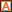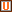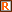## Precalculus Algebra

Course Course Title Class Lab/ Shop Clinical/ Co-op Credit
MAT 171 Precalculus Algebra3 2 0 4
Prerequisites: MAT 003 or MAT 121
Corequisites: MAT 071
Effective Term: Fall 2020
 This course is designed to develop topics which are fundamental to the study of Calculus. Emphasis is placed on solving equations and inequalities, solving systems of equations and inequalities, and analysis of functions (absolute value, radical, polynomial, rational, exponential, and logarithmic) in multiple representations. Upon completion, students should be able to select and use appropriate models and techniques for finding solutions to algebra-related problems with and without technology. This course has been approved for transfer under the Comprehensive Articulation Agreement as a general education course in Mathematics.
 Competencies ·Student Learning Outcomes·1. Use analytical, graphical, and numerical representations to solve absolute value, radical, polynomial, rational, exponential, and logarithmic equations with both real and complex solutions.·2. Use analytical, graphical, and numerical representations to solve absolute value, polynomial and rational inequalities with real solutions.·3. Use analytical, graphical, and numerical representations to analyze absolute value, radical, polynomial, rational, exponential and logarithmic functions with both real and complex zeros.·4. Use multiple methods to solve problems involving systems of equations and apply to decomposing partial fractions.·5. Construct the composition and inverse of functions.·6. Use polynomial, exponential and logarithmic functions to model various real world situations in order to analyze, draw conclusions, and make predictions.

MAT 003 Tier 3 Required

2013FA - New State Prereq: (DMA 010 and DMA 020 and DMA 030 and DMA 040 and DMA 050 and DMA 060 and DMA 070 and DMA 080)

2014FA - New Course Version (S23934)

2014FA - Identified Course as UGETC

2018FA – New Course Version (S24997)

2019SP - Revised State Prereq: Add MAT 003 and Local Coreq: Add MAT 071

2020FA – New Course Version (S25432)

Legend

• : Comprehensive Articulation Agreement - This course is recommended for students transferring to a four-year university.
• : Local Requirement
• : Instructional Service Agreement
• : Universal General Education Transfer Component (UGETC)
• : Global Distinction
• : Requisite(s)